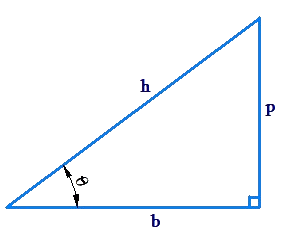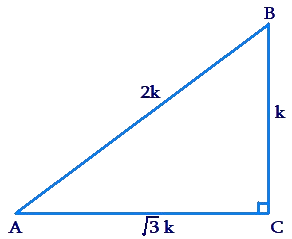# Basic Properties of Trigonometric Ratios

Basic Properties of Trigonometric Ratios

## Reciprocal relations:

•   \begin{align}\sin \theta = \frac{1}{{\rm{cosec}\;\theta }}\,\,;\,\sin \theta \,\rm{cosec}\;\theta = 1\end{align}

•   \begin{align}\cos \theta = \frac{1}{{\sec \theta }}\,\,;\,\cos \theta \,\sec \theta = 1\end{align}

•   \begin{align}\tan \theta = \frac{1}{{\cot \theta }}\,;\,\tan \theta \,\cot \theta = 1\end{align}

We also note the following:

• \begin{align}\tan \theta = \frac{p}{b} =\frac{\frac{p}{h}}{\frac{b}{h}} = \frac{{\sin \theta }}{{\cos \theta }}\end{align}
• \begin{align}\cot \theta = \frac{1}{{\tan \theta }} = \frac{{\cos \theta }}{{\sin \theta }}\end{align}

✍Note: We can write all of $$\tan \theta ,\;\cot \;\theta, \rm{cosec}\; \theta$$ and $$\sec \theta$$ in terms of $$\sin \theta$$ and $$\cos \theta$$.

The next set of relations is extremely important, which can be formularized by using Pythagoras Theorem and Trigonometric ratios, called Trigonometric Identities.

## Trigonometric Identities:

An equation is called an identity when it is true for all values of the variables involved. Similarly, an equation involving trigonometric ratios of an angle is called a trigonometric identity, if it is true for all values of the angles involved.
There are three improtant trigonometric identities which are extensively used throughout the topic of trigonometry. These are as follows:

1.  $${\sin ^2}\;\theta + {\cos ^2}\theta = 1$$
2. $$1 + {\tan ^2}\theta = {\sec ^2}\theta$$
3. $$1 + {\cot ^2}\theta = {{\mathop{\rm cosec}\nolimits} ^2}\theta$$

Proof: Consider the following right angled-triangle:By using Pythagoras Theorem:

1. $${p^2} + {b^2} = {h^2}$$
\begin{align} &\Rightarrow \frac{{{p^2}}}{{{h^2}}} + \frac{{{b^2}}}{{{h^2}}} = 1\\&\Rightarrow {\left( {\frac{p}{h}} \right)^2} + {\left( {\frac{b}{h}} \right)^2} = 1\\&\Rightarrow \boxed {{\sin ^2}\theta + {\cos ^2}\theta = 1}\end{align}
2. $${p^2} + {b^2} = {h^2}$$
\begin{align}&\Rightarrow \frac{{{p^2}}}{{{b^2}}} + 1 = \frac{{{h^2}}}{{{b^2}}}\\&\Rightarrow 1 + {\left( {\frac{p}{b}} \right)^2} = {\left( {\frac{b}{h}} \right)^2}\\ &\Rightarrow \boxed {1 + {\tan ^2}\theta = {\sec ^2}\theta} \end{align}
3. $${p^2} + {b^2} = {h^2}$$
\begin{align}&\,\, \Rightarrow 1 + \frac{{{b^2}}}{{{p^2}}} = \frac{{{h^2}}}{{{p^2}}}\\&\,\, \Rightarrow 1 + {\left( {\frac{b}{p}} \right)^2} = {\left( {\frac{h}{b}} \right)^2}\\&\,\, \Rightarrow \boxed {1 + {\cot ^2}\theta = \rm{cosec}{^2}\theta} \end{align}

We see that these relations follow simply from the Pythagoras Theorem. Nevertheless, they are extremely important, used very extensively and should be committed to memory.

## Solved Examples:

Example 1: It is given that $$\sec \theta \,\rm{equals}\,\frac{{25}}{7}$$ . Find the other trigonometric ratios for $$\theta$$.

Solution: We have:

1. \begin{align}\cos \theta = \frac{1}{{\sec \theta }} = \boxed {\frac{7}{{25}}}\end{align}
2. $$\sin \theta = \sqrt {1 - {{\cos }^2}\theta }$$
\begin{align}\\&\,\,\,\,\,\,\,\,\,\,\, = \sqrt {1-\frac{{49}}{{625}}} \\&\,\,\,\,\,\,\,\,\,\,\, = \sqrt {\frac{{576}}{{625}}} = \boxed {\frac{{24}}{{25}}}\end{align}

3. \begin{align}\tan \theta = \frac{{\sin \theta }}{{\cos \theta }} = =\frac{\frac{24}{25}}{\frac{7}{25}}= \boxed {\frac{{24}}{7}}\end{align}

4. \begin{align}\rm{cosec}\;\theta = \frac{1}{{\sin \theta }} = \boxed {\frac{{25}}{{24}}}\end{align}

5. \begin{align}\cot \theta = \frac{1}{{\tan \theta }} = \boxed {\frac{7}{{24}}}\end{align}

✍Note: This example shows that knowing one trigonometric ratios for a particular angle enables us to determine all the other trigonometric ratios for that angle.

Example 2: If \begin{align}\sin\theta = \frac{4}{5},\end{align} find the value of \begin{align}\left( {\frac{{\sec \theta + \cot \theta }}{{5\;\rm{cosec} - 4\tan \theta }}} \right)\end{align}.

Solution: We have,

\begin{align}&\cos \theta = \sqrt {1 - {{\sin }^2}\theta } = \frac{3}{5}\\&\sec \theta = \frac{1}{{\cos \theta }} = \frac{5}{3}\\&\tan \theta = \frac{{\sin \theta }}{{\cos \theta }} = \frac{4}{5}\\&\cot \theta = \frac{1}{{\tan \theta }} = \frac{3}{4}\\&\rm{cosec}\;\theta = \frac{1}{{\sin \theta }} = \frac{5}{4}\end{align}

If we denote the expression in the question by $$E$$, we have:

\begin{align}&E = \left( {\frac{{\frac{5}{3} + \frac{3}{4}}}{{5 \times \frac{5}{4} - 4 \times \frac{4}{3}}}} \right)\\&\,\,\,\,\, = \frac{{\frac{{29}}{{12}}}}{{\frac{{11}}{{12}}}} = \boxed {\frac{{29}}{{11}}}\end{align}

Example 3: $$\Delta ABC$$ is right-angled at C, and \begin{align}\tan A = \frac{1}{{\sqrt 3 }}\end{align}. Find the value of $$\sin A \cos B + \cos A\sin B$$.

Solution: Consider the following figure:Note how we have used the fact that \begin{align}\tan A = \frac{1}{{\sqrt 3 }}\end{align} and the Pythagoras theorem to write the lengths of the triangle sides in term of some $$k > 0$$.

Now,

\begin{align}&\sin A = \frac{1}{2},\;\cos A = \frac{{\sqrt 3 }}{2}\\&\sin B = \frac{{\sqrt 3 }}{2},\;\cos B = \frac{1}{2}\end{align}

Thus,

\begin{align}&\sin A\cos B + \cos A\sin B\\& = \frac{1}{2} \times \frac{1}{2} + \frac{{\sqrt 3 }}{2} \times \frac{{\sqrt 3 }}{2}\\&\Rightarrow \frac{1}{4} + \frac{3}{4} = \boxed {1}\end{align}

Challenge: It is given that $$\sin \theta = \frac{5}{7}$$. Find the other trigonometric ratios for $$\theta$$.

⚡Tip: Use a similar approach as in example-1.

More Important Topics
More Important Topics
Learn from the best math teachers and top your exams

• Live one on one classroom and doubt clearing
• Practice worksheets in and after class for conceptual clarity
• Personalized curriculum to keep up with school